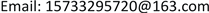1. 引言

2. 第三方海外仓储费用构成分析

3. 跨境电商第三方海外仓储成本模型3.1. 头程运输成本C<sub>1</sub>

c11：一次订购费用。

c12：包括存储费用和出入库操作服务费用。根据单件货物体积和存储时间，按照一定费率收取的仓库存储和服务费用。

{ c 12 = 0 + m o + n o × Q × g o                                                         t 1 ≤ t 0 1 ;     t 0 1 ≥ 0 c 12 = Q × K i 1 × ( t 1 − t 0 1 ) + m o + n o × Q × g o                 V i − 1 ≤ v o ≤ V i (1)

t 0 1 ：境内转运仓免仓租期(单位：天)；

t1：境内转运仓商品储存时间(单位：天)；

Q：订货批量；

Vi：境内转运仓单件货物体积计费区间上限；

Vi−1：境内转运仓单件货物体积计费区间下限；

K i 1 ：单件货物体积在 V i − 1 ≤ V 0 ≤ V i 区间之内的境内转运仓仓租费费率；

mo：境内转运仓入库作业费用常数；

no：境内转运仓每公斤货物入库作业费用；

go：单件货物重量。

c13：境内运输一般采用快递的方式，运输费用按照首重和续重的计费标准计费：

c 13 = c o + h o × Q × g o (2)

co：从采购地到境内转运仓的首重费用；

ho：从采购地到境内转运仓的每公斤货物续重费用；

go：为单件货物重量。

c14：境内转运仓库到海外仓库的运输费用，根据货物的总体积的大小，采用不同的计算费率：

c 14 = Q × V o × R i ,         V 1 + i 1 ≥ Q × V o ≥ V i 1 (3)

v0：单位产品体积；

V 1 + i 1 ：单件货物海运运费计费区间上限体积；

V i 1 ：单件货物海运运费计费区间下限体积；

Ri：一批货物体积数量在 V 1 + i 1 ≥ Q × V o ≥ V i 1 区间内的每立方米货物海运运费。

C 1 = c 1 × D Q (4)

3.2. 综合关税C<sub>2 </sub>

C 2 = e o × D Q (5)

eo：每次清关总费用。

3.3. 第三方海外仓储服务成本C<sub>3 </sub>

{ C 3 = 0 × ( 1 2 × Q + S )                                                   t 2 ≤ t 0 2 ;     t 0 2 ≥ 0 C 3 = M i 2 × ( t 2 − t 0 2 ) × ( 1 2 × Q + S )                   t 2 > t 0 2 ;     V i − 1 2 ≤ v o ≤ V i 2 (6)

M i 2 ：表示海外仓仓租费率；

t 0 2 ：表示海外仓面仓租期(单位：天)；

V i 2 ：表示单件货物海外仓存储费计费区间上限体积；

V i − 1 2 ：表示单件货物海外仓存储费计费区间下限体积。

3.4. 缺货损失C<sub>4</sub>

Y = D Y + S = μ × ω + S (7)

F x ( d ) = e − x ( x ) d d ! (8)

Q Y = ∑ d = Y + 1 ∞ ∑ x = μ − ε μ + ε P ( x ) × F x ( d ) × d (9)

C 4 = D Q × f × Q Y (10)

3.5. 总成本C

C = C 1 + C 2 + C 3 + C 4 (11)

4. 实证分析

4.1. 头程运输成本

C 1 = 148 × D Q + 4.4 × D

4.2. 综合关税

C 2 = e o × D Q = 72 × D Q

4.3. 海外仓存储服务费用

C 3 = 16.5 × ( 1 2 × Q + S )

4.4. 缺货损失

P ( x ) = 1 2 π σ e − ( x − 20 ) 2 / 2

Q Y = ∑ d = Y + 1 Y + 15 ∑ x = 18 22 P ( x ) × F x ( d ) × d

C 4 = D Q × 250 × Q Y

C = C 1 + C 2 + C 3 + C 4 = ( 148 + 72 + 250 × Q Y ) × D Q + 8.25 × Q + 4.4 × D + 16.5 × S

Calculation table of order point, safety stock, best order quantity, and cos
YY = 27Y = 29Y = 31Y = 36Y = 37Y = 38Y = 39
S791116171819
QY1.6430.7520.3090.0210.0110.0060.003
Q165.90133.43113.8999.1498.5998.3198.15

5. 结论